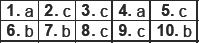# Probability-MCQ

Multiple Choice Questions
Probability
(1)   The probability of a leap year selected at random contain 53 Sunday is:
(a) 53/ 366
(b) 1/7
(c) 2/7
(d) 53/365
(2)   A bag contains 3 red and 2 blue marbles. A marble is drawn at random. The probability of drawing a black ball is :
(a) 3/5
(b) 2/5
(c) 0/5
(d) 1/5
(3)   The probability that it will rain tomorrow is 0.85. What is the probability that it will not rain tomorrow
(a) 0.25
(b) 0.145
(c) 3/20
(d) none of these
(4)   What is the probability that a number selected from the numbers (1, 2, 3,..........,15) is a multiple of 4?
(a) 1/5
(b) 4/5
(c) 2/15
(d) 1/3
(5)   What are the total outcomes when we throw three coins?
(a) 4
(b) 5
(c) 8
(d) 7
(6)   The probability that a prime number selected at random from the numbers (1,2,3, ..........35) is :
(a) 12/35
(b) 11/35
(c) 13/35
(d) none of these
(7)   The sum of the probability of an event and non event is :
(a) 2
(b) 1
(c) 0
(d) none of these.
(8)   The following probabilities are given; choose the correct answer for that which is not possible.
(a) 0.15
(b) 2/7
(c) 7/5
(d) none of these.
(9)   If three coins are tossed simultaneously, than the probability of getting at least two heads, is
(a) 1/4
(b) 3/8
(c) 1/2
(d) 1/8
(10)   A letter is chosen at random from the letters of the word �ASSASSINATION�. The probability that the letter chosen has:
(a) 6/13
(b) 7/13
(c) 1
(d) none of these.